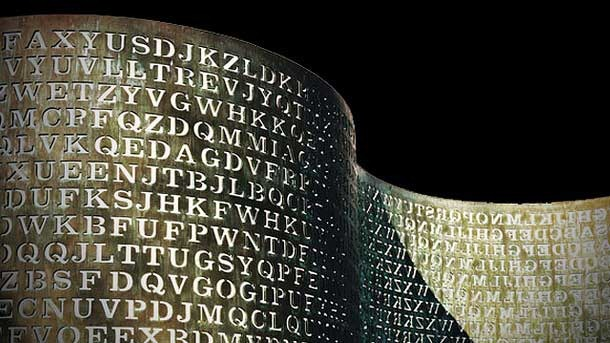# Java Symmetric Encryption Decryption using Java Cryptography Extension (JCE)

This tutorial is to demonstrate how to encrypt and decrypt in Java using the Java Cryptography Extension (JCE). Symmetric key and asymmetric key are the two basic types of cryptographic systems. They are also called as “secret key” and “public key” cryptography.

One of the success factors to Java is attributed to the strong security it provides to the platform and applications. This is the first part of a series of tutorials on Java security I am about to write. In this tutorial, we will see a simple example on using the “secret key” cryptography with JCE.Security and its implementation is one of the difficult areas for programmers. I will be writing on basic stuff and as always JavaPapers focus group is beginners and intermediate. So do not stay away from this series, there is lot of interesting things to learn.

## Symmetric Key Cryptography

Symmetric (secret) key uses the same key for encryption and decryption. The main challenge with this type of cryptography is the exchange of the secret key between the two parties sender and receiver.

In the following example we will use the encryption and decryption algorithm available as part of the JCE SunJCE provider.

## Symmetric Key Java Encryption Decryption Example

The following example uses symmetric key for encryption and decryption. “Data Encryption Standard (DES)” was a popular symmetric key algorithm. Presently DES is outdated. DESede is a triple DES and a stronger variant of DES.

1. Add the Security Provider. We are using the SunJCE Provider that is available with the JDK.
2. Generate Secret Key. Use KeyGenerator and an algorithm to generate a secret key. We are using DESede. There are other algorithms like blowfish.
3. Encode Text. For consistency across platform encode the plain text as byte using UTF-8 encoding.
4. Encrypt Text. Instantiate Cipher with ENCRYPT_MODE, use the secret key and encrypt the bytes.
5. Decrypt Text. Instantiate Cipher with DECRYPT_MODE, use the same secret key and decrypt the bytes.

AES is the latest encryption standard over the DES. You can refer the encryption decryption with AES symmetric algorithm using JCE tutorial. All the above given steps and concept are same, we just replace the DES with AES.

```package com.javapapers.java.security;

import java.security.Security;

import javax.crypto.Cipher;
import javax.crypto.KeyGenerator;
import javax.crypto.SecretKey;

public class Encryption {
private static Cipher cipher = null;

public static void main(String[] args) throws Exception {

// uncomment the following line to add the Provider of choice

KeyGenerator keyGenerator = KeyGenerator.getInstance("DESede");
// keysize must be equal to 112 or 168 for this provider
keyGenerator.init(168);
SecretKey secretKey = keyGenerator.generateKey();
cipher = Cipher.getInstance("DESede");

String plainText = "Java Cryptography Extension";
System.out.println("Plain Text Before Encryption: " + plainText);

byte[] plainTextByte = plainText.getBytes("UTF8");
byte[] encryptedBytes = encrypt(plainTextByte, secretKey);

String encryptedText = new String(encryptedBytes, "UTF8");
System.out.println("Encrypted Text After Encryption: " + encryptedText);

byte[] decryptedBytes = decrypt(encryptedBytes, secretKey);
String decryptedText = new String(decryptedBytes, "UTF8");
System.out.println("Decrypted Text After Decryption: " + decryptedText);
}

static byte[] encrypt(byte[] plainTextByte, SecretKey secretKey)
throws Exception {
cipher.init(Cipher.ENCRYPT_MODE, secretKey);
byte[] encryptedBytes = cipher.doFinal(plainTextByte);
return encryptedBytes;
}

static byte[] decrypt(byte[] encryptedBytes, SecretKey secretKey)
throws Exception {
cipher.init(Cipher.DECRYPT_MODE, secretKey);
byte[] decryptedBytes = cipher.doFinal(encryptedBytes);
return decryptedBytes;
}
}```

### Example Program Output

```Plain Text Before Encryption: Java Cryptography Extension
Decrypted Text After Decryption: Java Cryptography Extension```

### Access restriction Error

I am using Java SE 8 and Eclipse to run the above program. Pre Java 1.4 you need to add the SunJCE provider explicitly and you may get the following compilation error,

Access restriction: The constructor SunJCE() is not accessible due to restriction on required library C:\Program Files\Java\jdk1.8.0\jre\lib\ext\sunjce_provider.jar

To solve this, remove the JRE system library and re add the same. Go to “Java Build Path” via Project Properties, then under Libraries tab, remove JRE System Library and again add the same back.

## Comments on "Java Symmetric Encryption Decryption using Java Cryptography Extension (JCE)"

1. […] decryption using Java Cryptography Extension (JCE). In the previous tutorial we saw about encryption decryption using DES symmetric key algorithm. “Data Encryption Standard (DES)” is prone to brute-force attacks. It is a old way of […]

2. ashwin chauhan says:

good!

3. Divya says:

package com.javapapers.java.security;
Where does the package exist??

4. Dejega says:

So how do we exchange the secret key between the two parties involved, i.e. sender and receiver ?

Comments are closed for "Java Symmetric Encryption Decryption using Java Cryptography Extension (JCE)".